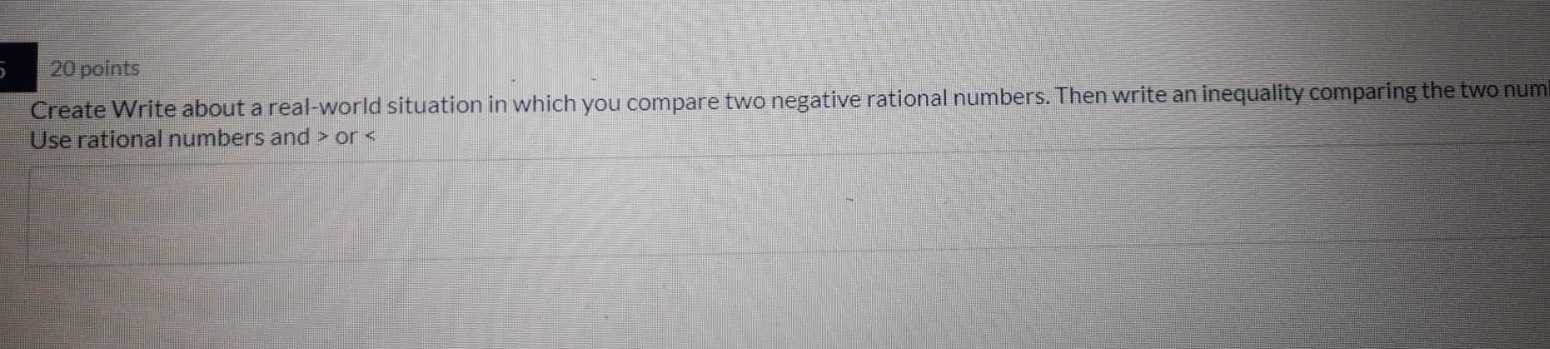### ¿Todavía tienes preguntas de matemáticas?

Pregunte a nuestros tutores expertos
Algebra
Pregunta$$20$$ points Create Write about a real-world situation in which you compare two negative rational numbers. Then write an inequality comparing the two num Use rational numbers and $$>$$ or $$<$$